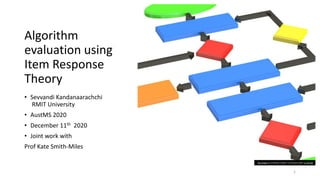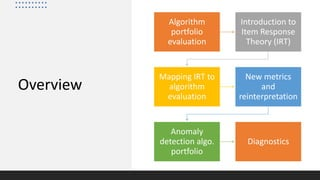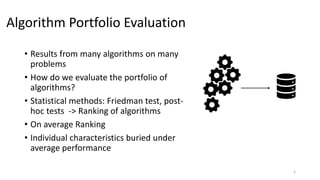Successfully reported this slideshow.

# Algorithm evaluation using item response theory

0

Share×
1 of 28
1 of 28

# Algorithm evaluation using item response theory

0

Share

Item Response Theory (IRT) is a paradigm within the field of Educational Psychometrics, that is used to assess student ability and test question difficulty and discrimination power. IRT has recently been applied to evaluate
machine learning algorithm performance on a classification dataset. Here, we present a modified IRT-based framework for evaluating a portfolio of algorithms across a repository of datasets, while eliciting a suite of richer characteristics such as stability, effectiveness and anomalousness, that describe different aspects of algorithm performance.

Item Response Theory (IRT) is a paradigm within the field of Educational Psychometrics, that is used to assess student ability and test question difficulty and discrimination power. IRT has recently been applied to evaluate
machine learning algorithm performance on a classification dataset. Here, we present a modified IRT-based framework for evaluating a portfolio of algorithms across a repository of datasets, while eliciting a suite of richer characteristics such as stability, effectiveness and anomalousness, that describe different aspects of algorithm performance.

## More Related Content

### Related Books

Free with a 30 day trial from Scribd

See all

### Related Audiobooks

Free with a 30 day trial from Scribd

See all

### Algorithm evaluation using item response theory

1. 1. Algorithm evaluation using Item Response Theory • Sevvandi Kandanaarachchi RMIT University • AustMS 2020 • December 11th 2020 • Joint work with Prof Kate Smith-Miles 1 This Photo by Unknown Author is licensed under CC BY-ND
2. 2. Overview Algorithm portfolio evaluation Introduction to Item Response Theory (IRT) Mapping IRT to algorithm evaluation New metrics and reinterpretation Anomaly detection algo. portfolio Diagnostics
3. 3. Algorithm Portfolio Evaluation • Results from many algorithms on many problems • How do we evaluate the portfolio of algorithms? • Statistical methods: Friedman test, post- hoc tests -> Ranking of algorithms • On average Ranking • Individual characteristics buried under average performance 3
4. 4. Item Response Theory • Latent trait models used in social sciences/psychometrics • Unobservable characteristics and observed outcomes • Verbal or mathematical ability • Racial prejudice or stress proneness • Political inclinations • Intrinsic “quality” that cannot be measured directly This Photo by Unknown Author is licensed under CC BY-SA
5. 5. IRT in education • 𝑁 Students (participants) answer 𝑛 questions (test item) • Student ability (latent trait continuum) • Test item discrimination • Test item difficulty This Photo by Unknown Author is licensed under CC BY 5
6. 6. Dichotomous IRT • Multiple choice • True or false • 𝜙 𝑥𝑖𝑗 = 1 𝜃𝑖, 𝛼𝑗, 𝑑𝑗, 𝛾𝑗 = 𝛾𝑗 + 1 −𝛾 𝑗 1+exp(−𝛼 𝑗(𝜃 𝑖−𝑑 𝑗)) • 𝑥𝑖𝑗 - outcome/score of examinee 𝑖 for item 𝑗 • 𝜃𝑖 - examinee’s (𝑖) ability • 𝛾𝑗 - guessing parameter for item 𝑗 • 𝑑𝑗 - difficulty parameter • 𝛼𝑗 - discrimination This Photo by Unknown Author is licensed under CC BY-NC 6
7. 7. Polytomous IRT • Letter grades • Score out of 5 • Theta is the ability • For each score there is a curve • 𝑃(𝑥𝑖𝑗 = 𝑘|𝜃𝑖, 𝑑𝑗, 𝛼𝑗) • For a given ability what's the score you’re most likely to get 7
8. 8. Continuous IRT • Grades out of 100 • A 2D surface of probabilities • 𝑃(𝑧𝑖𝑗|𝜃𝑖, 𝑑𝑗, 𝛼𝑗) 8
9. 9. Mapping algorithm evaluation to IRT • Item characteristics • Difficulty, discrimination • Person characteristic • Ability • In traditional IRT • examinees > > questions IRT Model Person-doing something Test - inanimate 9
10. 10. Mapping IRT to algorithm evaluation (Standard) • Dataset (item) characteristics • Difficulty, discrimination • Algorithm (person) characteristic • Ability • We are evaluating datasets more than algorithms! IRT Model Algorithm-doing something Dataset - inanimate 10
11. 11. New Inverted Mapping • Dataset (person) characteristic • Person ability dataset easiness • Algorithm (item) characteristics • Item difficulty algo. easiness threshold • Item discrimination algo stability, and anomalousness • Now we are evaluating algorithms more than datasets. IRT Model Algorithm-doing something Dataset - inanimate 11
12. 12. What are these new parameters? • IRT - 𝜃𝑖 - ability of examinee 𝑖 • 𝜃 increases probability of a higher score increases • What is 𝜃𝑖, in terms of a dataset? • 𝜃𝑖 - easiness of the dataset 12
13. 13. What are these new parameters? • IRT - 𝛼𝑗- discrimination of item 𝑗 • 𝛼𝑗increases → slope of curve increases • What is 𝛼𝑗, in terms of an algorithm? • 𝛼𝑗- lack of stability/robustness of algo • (1/|𝛼 𝑗|)- stability/robustness of algo 13
14. 14. Stable algorithms • Education – such a question doesn’t give any information • Algorithms – these algorithms are really stable • Stability = 1/|𝛼𝑗| 14
15. 15. Anomalous algorithms • Algorithms that perform poorly on easy datasets and well on difficult datasets • Negative discrimination • In education – such items are discarded or revised • If an algorithm anomalous, it is interesting • Anomalousness = sign(𝛼𝑗) This Photo by Unknown Author is licensed under CC BY-NC-ND 15
16. 16. Fitting Continuous IRT models • Continuous models • Does not fit items (algorithms) with negative discrimination • d • 𝛼𝑗 - discrimination parameter, 𝛾𝑗 - scaling parameter (for this formulation). . . Assumption 𝛼𝑗 > 0, 𝛾𝑗 > 0 • 𝐶𝑗 - Covariance term • 𝑡 - the iteration • Negative covariance stops convergence 16 Minimize this Variance term
17. 17. Fitting continuous IRT models 17 • Probability of score, given the ability • Works if both 𝛼𝑗 > 0, 𝛾𝑗 > 0 OR 𝛼𝑗 < 0 , 𝛾𝑗 < 0 → 𝑠𝑖𝑔𝑛 𝛼𝑗 = 𝑠𝑖𝑔𝑛 𝛾𝑗 • So modify the original assumption 𝛼𝑗 > 0, 𝛾𝑗 > 0 to 𝑠𝑖𝑔𝑛 𝛼𝑗 = 𝑠𝑖𝑔𝑛 𝛾𝑗
18. 18. Anomaly detection (8 algos, 3142 datasets) 18
19. 19. What about the latent trait? (dataset easiness spectrum) 19
20. 20. Dataset easiness and algorithm performance 20
21. 21. Dataset easiness and algorithm performance 21
22. 22. Dataset easiness and algorithm performance 22 Latent trait occupancy! How much latent trait do you occupy?
23. 23. Diagnostics 23
24. 24. How well does the IRT model fit? • Difference 𝑦𝑖𝑗 = |𝑥𝑖𝑗 − ො𝑥𝑖𝑗| • Cumulative distribution of these differences • 𝑃(𝑦𝑖𝑗 ≤ 𝑐) for different 𝑐 • Model goodness curve (MGC) • Area under this curve (AUMGC) • Higher AUMGC is better • Same idea for polytomous and continuous 24
25. 25. Effectiveness of algorithms • Effective algorithms give better performances for most datasets • 𝑃 𝑥𝑖𝑗 ≥ 𝑐 - Actual • 𝑃 ො𝑥𝑖𝑗 ≥ 𝑐 - Predicted • Area under these curves • Area Under Actual Effectiveness Curve (AUAEC) • Area Under Predicted Effectiveness Curve (AUPEC) 25
26. 26. Actual and Predicted effectiveness • We can plot (AUAEC, AUPEC) as well. 26
27. 27. Summary • Evaluating a portfolio of algorithms • Use Item Response Theory from Psychometrics • Accommodating it to include negative discrimination • Inverting the intuitive mapping -> elegant reinterpretation • A richer understanding of algorithms • Includes additional diagnostics to test the goodness of the IRT model • R package airt (on CRAN) • https://sevvandi.github.io/airt/ • Pre-print: http://bit.ly/algorithmirt • Comprehensive Algorithm Portfolio Evaluation using Item Response Theory • More applications included 27
28. 28. 28